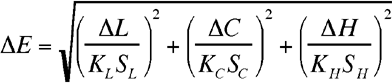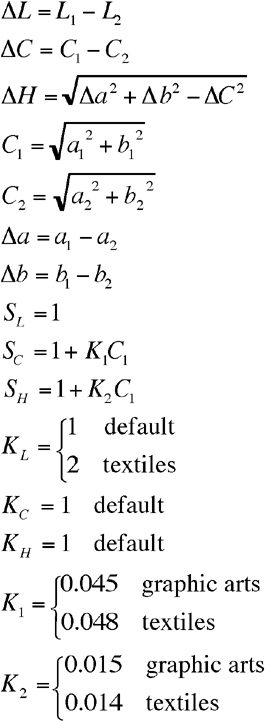# Color difference algorithm – part 2

It’s time to see how to calculate difference between two colors.

I am using the CIE1994 formula because it’s quite accurate and not that complex to calculate.

Here it is, assuming I have two colors in Lab formatwhere(thanx to Bruce Lindbloom)

First, I am turning the scripts explained at Color difference algorithm into functions, this way:

```function rgb_to_xyz(\$rgb){
\$red = \$rgb;
\$green = \$rgb;
\$blue = \$rgb;
\$_red = \$red/255;
\$_green = \$green/255;
\$_blue = \$blue/255;
if(\$_red>0.04045){
\$_red = (\$_red+0.055)/1.055;
\$_red = pow(\$_red,2.4);
}
else{
\$_red = \$_red/12.92;
}
if(\$_green>0.04045){
\$_green = (\$_green+0.055)/1.055;
\$_green = pow(\$_green,2.4);
}
else{
\$_green = \$_green/12.92;
}
if(\$_blue>0.04045){
\$_blue = (\$_blue+0.055)/1.055;
\$_blue = pow(\$_blue,2.4);
}
else{
\$_blue = \$_blue/12.92;
}
\$_red *= 100;
\$_green *= 100;
\$_blue *= 100;
\$x = \$_red * 0.4124 + \$_green * 0.3576 + \$_blue * 0.1805;
\$y = \$_red * 0.2126 + \$_green * 0.7152 + \$_blue * 0.0722;
\$z = \$_red * 0.0193 + \$_green * 0.1192 + \$_blue * 0.9505;
return(array(\$x,\$y,\$z));
}```

and…

```function xyz_to_lab(\$xyz){
\$x = \$xyz;
\$y = \$xyz;
\$z = \$xyz;
\$_x = \$x/95.047;
\$_y = \$y/100;
\$_z = \$z/108.883;
if(\$_x>0.008856){
\$_x = pow(\$_x,1/3);
}
else{
\$_x = 7.787*\$_x + 16/116;
}
if(\$_y>0.008856){
\$_y = pow(\$_y,1/3);
}
else{
\$_y = (7.787*\$_y) + (16/116);
}
if(\$_z>0.008856){
\$_z = pow(\$_z,1/3);
}
else{
\$_z = 7.787*\$_z + 16/116;
}
\$l= 116*\$_y -16;
\$a= 500*(\$_x-\$_y);
\$b= 200*(\$_y-\$_z);
return(array(\$l,\$a,\$b));
}```

Now, it’s time to determine color difference, using the above formula:

```function de_1994(\$lab1,\$lab2){
\$c1 = sqrt(\$lab1*\$lab1+\$lab1*\$lab1);
\$c2 = sqrt(\$lab2*\$lab2+\$lab2*\$lab2);
\$dc = \$c1-\$c2;
\$dl = \$lab1-\$lab2;
\$da = \$lab1-\$lab2;
\$db = \$lab1-\$lab2;
\$dh = sqrt((\$da*\$da)+(\$db*\$db)-(\$dc*\$dc));
\$first = \$dl;
\$second = \$dc/(1+0.045*\$c1);
\$third = \$dh/(1+0.015*\$c1);
return(sqrt(\$first*\$first+\$second*\$second+\$third*\$third));
}```

And you’ll find the difference between colors.

Next time, a real application using all this boring theory… (you will be surprised…)

214 GAME PROTOTYPES EXPLAINED WITH SOURCE CODE
// 1+2=3
// 10000000
// 2 Cars
// 2048
// Avoider
// Ballz
// Block it
// Blockage
// Bloons
// Boids
// Bombuzal
// Breakout
// Bricks
// Columns
// CubesOut
// Dots
// DROP'd
// Dudeski
// Eskiv
// Filler
// Fling
// Globe
// HookPod
// Hundreds
// InkTd
// Iromeku
// Lumines
// Magick
// MagOrMin
// Maze
// Memdot
// Nano War
// Nodes
// o:anquan
// Ononmin
// Pacco
// Phyballs
// Platform
// Poker
// Pool
// Poux
// Pudi
// qomp
// Racing
// Renju
// SameGame
// Security
// Sling
// Slingy
// Sokoban
// Splitter
// Sproing
// Stack
// Stringy
// Sudoku
// Tetris
// Threes
// Toony
// Turn
// TwinSpin
// vvvvvv
// Wordle
// Worms
// Yanga
// Zhed
// zNumbers